# All about Gear Effect - 4

Dave Tutelman  --  February 20, 2009

## Does shaft tip stiffness limit vertical gear effect?

It had never really occurred to me to investigate this. After all, my previous studies were pretty clear that:
Why would I expect this answer to come out differently?

Then, over the last couple of months, three different people pointed me to a 3-year-old post by Dana Upshaw on Tom Wishon's forum. Dana wrote:
I sure can't come up with a better explanation of the results than:
1. Vertical gear effect can have a significant effect on driver backspin. (But we knew that a few pages ago).
2. The shaft can have a significant effect on vertical gear effect.
• Tom Wishon has posted that vertical gear effect is good for no more than 300-500rpm.
• Dana Upshaw has posted launch monitor data with a difference of 3300rpm between a top and a bottom hit (almost a 2" vertical difference) at less than 150mph ball speed.
Wishon and Upshaw both have tons of experience and good insight into what they are doing. So why the huge difference? And whom to believe? Well, we know from the analysis earlier in the article that numbers like Upshaw's -- and even higher -- are mathematically possible. If there is something substantially different between their experiments that is causing the discrepancies, it has to be something other than the usual suspects, like moment of inertia, ball speed, clubhead design, or the size or type of the miss. Even added together they don't account for as much as half the difference. Moreover, equation 3a -- our fundamental model of vertical gear effect -- held up very well against real measured data.

So let's investigate the explanation suggested by Dana's first post: the tip stiffness of the shaft.

The approach will be similar to what we did for shaft torque and horizontal gear effect. But it is harder this time. The steps:
1. Find the size of the vertical-plane torque applied to the head by the ball.
2. Find the amount of vertical rotation during impact, assuming no resistance provided by the shaft.
3. Find the amount of torque resistance the shaft could provide, given that amount of rotation. This is hard. There is no shaft specification comparable to the torque rating, to help us with this. Much of our investigation will necessarily be a search for this number.
4. See whether the shaft resistance induced by the rotation is a significant fraction of the torque we found in step #1 above. If not, then shaft stiffness cannot limit vertical gear effect.

### 1. Find the vertical-plane torque applied to the head by the ball.

This is no different from what we did before, except that we are now in a vertical plane. So:
Τavg  =  .771 yVb
The units are foot-pounds for torque, inches for y, and miles per hour for Vb.

### 2. Find the vertical rotation during impact, with no shaft resistance.

By the same reasoning:
 rotation  =  74.2 Vb y Iv
The units are degrees for rotation,  inches for y, miles per hour for Vb, and gram-centimeters-squared for Iv.

This time, we'd like to plot how we got to this total rotation. Assuming a constant moment (not accurate, but it will give us a reasonable approximation), the degrees of rotation during impact is a square-law curve based on the rotational analog of the well-known linear motion equation:
x = ½at2
We already know x (the rotation) at time t=.5msec; we can plug that in for x and solve for a:

 rotation  =  74.2 Vb y Iv =  ½a(0.5)2
 a  =  594 Vb y Iv
where t is in milliseconds. If we plug this back into ½at2, we get:
 rotation(t)  =  297 Vb Iv y t2 0 ≤ t ≤ 0.5 msec
We will use this later in step #4.

### 3. Find the amount of resistance to that rotation the shaft could provide.Figure 4-1
Now we get to the hard part. One of the things that makes it hard is that the clubhead can apply two contradictory loads at the tip of the shaft. Consider the case where the impact is high on the face. That generates a load (shown in Figure 4-1) which is a composite of:
• A force against the face, which tends to bend the tip of the shaft backward.
• A torque to point the clubhead face-up, which tends to bend the tip of the shaft forward.
How does the shaft resolve these conflicting demands? First let us examine the static case. We will do a classical analysis of a cantilever beam with both a point load and a separate moment (torque) at the tip. If you are interested in how we do the analysis, it is in the appendix. Below, we continue with the results of the analysis.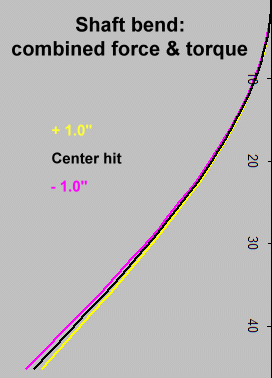Figure 4-2
For a shaft of length L and a uniform stiffness EI, we can find the shape of the deflection -- and, from that, the tip angle.
 Deflection at x = Vb 75.3 EI x2 ( y + x/3 -  L )
When this is differentiated to give the tip angle (and converted from radians to degrees), we find
 Tip Angle  = 1.52 Vb  EI L(y - L/2)
It is worth noting that the strength of the impact (given by ball speed Vb) and the stiffness of the shaft (EI) determine the size of the bend or the tip angle, but not the shape of the bend. They just scale the result.

Figure 4-2 is a picture plotted from the deflection equation: a collection of shaft bends as we vary how high the ball strikes the face. We use the typical length of a commercial driver, 45". Observations:
• A center-face hit (the black shaft) just bends the shaft backwards. This should be expected, because y=0 so the turning moment is zero. There is only a force pushing the head backward.
• They height of the hit on the face does not seem to matter much. The yellow and purple shafts represent hits a full inch above and below the center of the face, almost off the face and almost certainly well away from a point of good COR. Even so, this fairly extreme bending moment has very little effect on the shape of the bend. There is a little less backwards-downwards tilt for the high hit, but the difference is minimal.
• Here is an explanation for the very small difference. We know the moment exerted by the force of the ball on the clubhead (call it F) is Fy. But the bending moment exerted by F on the length of the shaft is F(L-x) at point x on the shaft. So, for most of the length of the shaft, the bending moment due to the F bending the whole shaft back is much more than the Fy moment. The result: the turning moment has a lot less effect than the backwards force in creating tip deflection.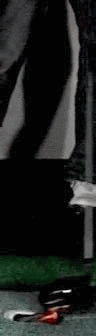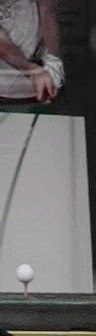Figure 4-3
But is that really how a shaft behaves?

Well... No! I asked Russ Ryden to capture impact and post-impact shaft behavior with his high-speed video camera. He loves this sort of stuff, and made a real project of it. We are still analyzing all the data, but here are a couple of videos that came out of Russ' efforts. They are slowed down even beyond the 1200 frames per second at which they were shot, so you can see them frame by frame. The frame interval is about 0.8 millisecond, or roughly twice the duration of impact.

The first video shows behavior at impact for a high-face hit with a shaft of medium tip stiffness. We see a lot more forward bend (moment-induced, not force-induced) than we would expect. But it occurs only near the tip, say within a foot of the clubhead. Moreover, it appears in the frame or two after impact (that is, the first millisecond or so after impact), and quickly vanishes. What is going on here?

The explanation becomes more clear when we look at the second video. There are two important differences: (i) the shaft is much more flexible, and (ii) it is close to a center hit, so all the bend is backwards. What we see is a wave of backwards bend starting near the clubhead and propagating up the shaft. The wave traverses the first two feet of the shaft in about four frames, or 3.3 milliseconds. So the speed of the wave up the shaft is about 0.6 foot/msec.

Thus our first static analysis that assumes the whole shaft is involved during impact is not a valid view, and not very useful for analysis purposes. If the wave travels at 0.6 foot/msec, only the bottom 4" of shaft tip can be involved in resisting clubhead rotation during impact. (Yes, a lot more of the shaft can be involved in all the clubhead rotation during the follow-through. But that does not influence ball flight; only head motion during impact can do that.)

The details of impact are very difficult to analyze without dynamic FEA software. But we can get a ballpark estimate of the ability of the shaft to resist rotation during impact. Let's look again at the static deflection formula, but this time limit the length to just the portion of the shaft that might be involved before the ball has left the clubface. That would be about 4" of shaft. Let's look at the shaft bend as the wave grows from 0" to 4" during impact. Here are shaft deflection curves for lengths of 1", 2", and 3".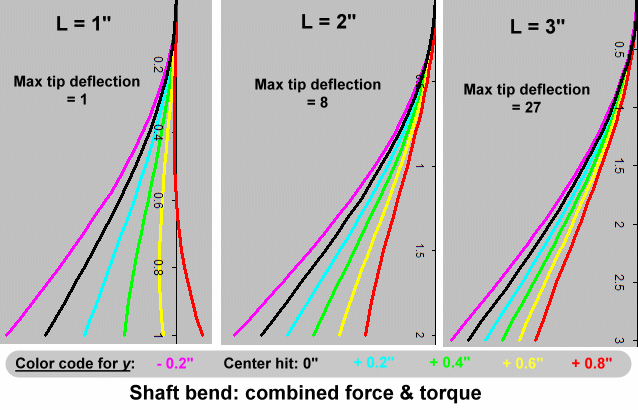Figure 4-4

Bear in mind that the graphs have been scaled so that detail will show. The 2" graph is really 8 times as wide as the 1" graph, and the 3" graph is 27 times as wide. That is because deflection grows rapidly as beam length increases; it is a cube-law relation.

The major conclusion I draw from Figure 4-4 is that, although the shaft might allow some upwards rotation early in the strike, the bend is all backwards for most of the duration of impact. By the time the ball releases from the clubface all the rotation is downward, and the differences in rotation due to height of impact are quite small.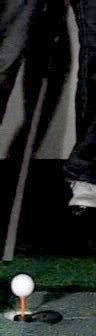t - 0.8msecImpactt + 0.8msec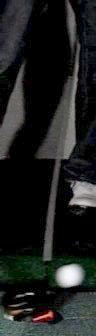t + 1.6msec
Figure 4-5

It's time to ask ourselves again, is that really how a shaft behaves?

We're closer now, but the answer is still no. Let's look again at Russ Ryden's videos. In Figure 4-5, we have taken snapshots of the first video -- the high-face hit -- in the vicinity of impact. If our analytical model were correct, then we might see a bit of sharp forward bend in the impact snapshot -- and only if it occurred early during the 0.5msec of impact. By the end of impact, the wave should have traveled 4" up the shaft and all the bend is backward. But what we actually see is a significant forward bend after the ball leaves the clubface. In fact, it is forward bend propagating up the shaft as the flex wave.

This is not at all what Figure 4-4 says will happen. That set of graphs, computed from the equations, predicts that any forward bend will disappear as the wave (the effective beam length) moves up the shaft during impact. By the time the ball releases from the clubface, all the bend is backward. But the snapshots in Figure 4-5 have a forward bend well after release!

What is wrong with the analytical model, and how can we fix it? The problem is that the model is still largely static. We have incorporated the flex wave to dynamically limit the length of shaft involved during impact. But we are still not accounting for the fact that some of the force and moment (P and M) are absorbed by the inertia of the clubhead and never get to the shaft at all, or at least not during impact. So the shaft sees less backward bend than P and less rotational moment than M. And the reduction may not be in equal proportion, because the mass (which resists P) is not the same as the moment of inertia (which resists M).

The way to resolve this is to separate out the response into independent fractions, as follows:
• ap is the fraction of the force absorbed by shaft flex.
• 1-ap is the fraction of the force absorbed by the clubhead's mass.
• am is the fraction of the moment absorbed by shaft flex.
• 1-am is the fraction of the moment absorbed by the clubhead's moment of inertia.
When we do that, the equations for deflection and tip angle become:
 Deflection at x = Vb 75.3 EI x2 ( amy + apx/3 -  apL )
 Tip Angle  = 1.52 Vb  EI L(amy - apL/2)Figure 4-6
Again, let's plot the shape of the shaft (the deflection curve). This time, we will try to come up with a shape similar to the bend we see in Figure 4-5 at t+0.8msec, immediately after impact. The photos show a very high-face hit and about a 6" flex wave, so we will use y=0.8" and L=6". We will vary am and ap. As it turns out, the shape depends on the ratio of am to ap and not their actual values (which determine only the magnitude of the curve, not the shape).

There is a plot in Figure 4-6 for values of am/ap from 2 to 50. It does not give a curve that looks remotely like Figure 4-5, the actual high-speed photo of the shaft tip, until am/ap is at least 10. So there isn't anything there suggesting the force (ap) being nearly as important as the moment (am) . I first plotted ratios from 0.1 to 10, but the values less than 5 didn't show forward bend at all. In order to look anything like Figure 4-5, we need a ratio of at least 10, and possibly more.

The conclusion we can draw from this is that, at least for Figure 4-5, the clubhead's mass absorbs so much of P that the shaft plays very little part in resisting the resulting backward bend. So just about all the deflection is forward bend due to M.

So we should use the equations as if ap is negligibly small compared to am. That gives us a shaft that looks something like Figure 4-5.
 Deflection at x = Vb 75.3 EI amx2y

 Tip Angle  = 1.52 Vb  EI amLy
The remaining prerequisite for relating clubhead rotation to shaft reaction is the EI of the shaft tip. I have an EI machine, and measured the EI of several known tip-stiff and tip-flexible shafts. My machine allows a 6" tip reading, which should be good enough; few shafts have much change of EI over the last 6" of tip.

I measured several shafts, some known tip-soft and others tip-stiff. For the units required by the equations, the lowest EI measured was just over 5200 pound-inches2 and the highest just under 14,000. (This corresponds to 14 and 40 N-m2 which is what the equations call for.) So tip stiffness covers nearly a 3:1 range. It is the range we will use in the next part of the analysis.

(Note: In practice, it would not be nearly as large as a 3:1 ratio. I got it with a tip-flexible LL-flex shaft and a tip-stiff long driver's XX-flex shaft. If you actually tried to fit a long drive competitor with an LL-flex shaft, the tip stiffness would be the least of your concerns. So, for a decent fit for a given golfer, the range of tip stiffness will be a lot less than 3:1. But let us continue with that ratio to keep things interesting.)

### 4. Compare shaft torque with impulse torque

Rather than compare torques directly, let's compute the amount of clubhead rotation when constrained:
1. Just by the moment of inertia.
2. Just by the shaft.
Then we will compare the rotations from #1 and #2. If the clubhead's inertia restricts rotation by an order of magnitude or two more than the shaft does, then the shaft has no effect on vertical gear effect, because the rotation never gets big enough during impact to put much load on the shaft.

In step #2, we found that the rotation during impact, restricted only by inertia, is given by:
 rotation  =  297 Vb Iv y t2 0 ≤ t ≤ 0.5
In step #3, we found the rotation (tip angle) of the shaft when subjected to the impulse moment of the ball is given by:
 Tip Angle  = 1.52 Vb  EI amLy
The beam length L starts at zero, and increases at a rate of 1.6 foot per millisecond, corresponding to the propagation of the flex wave.
Let's plot the rotation of the clubhead restricted by inertia and by shaft, and compare the plots. We will use the following values:
• Vb = 150mph, though that will not matter. Vb cancels out, because it is a proportional factor for both graphs.
• y = 0.8", though that also cancels out for the same reason.
• Iv = 3100 g-cm2.
• am = 1. We introduced am to reflect the fact that clubhead inertia restricts the shaft's role to some fraction. But the point of this plot is to see what happens if shaft flex is unrestricted by inertia, so we set am to 1.
• EI = 14 - 40 N-m2. We want to see how the effect of tip stiffness affects clubhead rotation, so we will plot the extremes of tip stiffness.
 Time (milliseconds) Inertial rotation (degrees) Beam length of flex wave (inches) Shaft Rotation (degrees) EI = 14 EI = 40 0 0 0 0 0 0.1 0.1 0.8 10.4 3.6 0.2 0.5 1.6 20.8 7.3 0.3 1.0 2.4 31.3 10.9 0.4 1.8 3.2 41.7 14.6 0.5 2.9 4.0 52.1 18.2

Now we compare how inertia limits the clubhead rotation (the yellow column) with how shaft torque limits it (the green columns). At every instant during impact, the shaft allows a lot more rotation than inertia does.
• For the tip-flexible shaft (EI=14), the shaft allows more than 18 times the rotation allowed by inertia, at every moment during impact. We can safely say that the shaft imposes no limit on the gear effect.
• For the tip-stiff shaft (EI=40), the numbers are more equivocal. The shaft still allows much more rotation than is allowed by inertia, but the factor is smaller. At its maximum influence just before release, the shaft allows 6 times the rotation that inertia does. That suggests that the shaft is absorbing about 14% of the moment, and inertia is absorbing 86%. Here is a table showing, moment by moment during impact, the relative loads absorbed by the shaft and the moment of inertia for a very tip-stiff shaft:  Milliseconds Absorbed by shaft Absorbed by inertia 0.1 3% 97% 0.2 6% 94% 0.3 8% 92% 0.4 11% 89% 0.5 14% 86%

### Conclusion

My conclusion from these numbers is that the tip stiffness of the shaft might make as much as a 14% difference in the spin due to vertical gear effect, but probably somewhat less and definitely not more than that.

I have mixed feelings about this conclusion. I believe it, but I have less confidence in it than the other results in this article. I went over the numbers many times (and found a few errors, mostly in unit conversions) before I was willing to post the article. Arguments for and against the conclusion:
• Against - It does not support Dana Upshaw's observation that tip stiffness can make a huge difference in vertical gear effect. The conclusion suggests a rather small difference. Explaining Dana's results would require a tip-stiff shaft to provide most of the rotation-limiting (not less than 14% as the calculations say). That also means that even a tip-flexible shaft should provide considerable limiting, since it will be at least 1/3 the tip stiffness of the stiffest shaft (and probably more than that, because the 3:1 ratio is not realistic).
• For - The numbers in my analysis which are most suspect are the shaft stiffness numbers. In order to get the sort of factor we would need to reconcile Upshaw's results, we would need the shaft stiffness to be much more limiting of the clubhead rotation than the inertia. That would require a total clubhead rotation during impact much less than inertia alone would allow, which is about 3º. But, measuring the high-speed photos in Figure 4-5, it looks like there is about a 3º head rotation during impact.  The rotation during impact roughly corresponds to inertial rotation -- and thus suggests that the shaft stiffness is not a major limiting factor.
•  pre- impact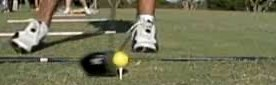post- impact+3.2 msec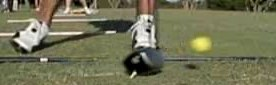+6.4 msec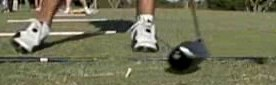+9.6 msecFigure 4-7
For - Consider another photo sequence from Russ Ryden's high-speed videos -- Figure 4-7. It doesn't show much shaft; instead, it follows the clubhead for nearly ten milliseconds after impact. Note how dramatically the head continues to rotate more and more face-up long after the ball has released -- and with it, any external moment trying to turn the head. What can we make of this?
We know from the analysis that the question -- does tip stiffness limit vertical gear effect? -- depends on the balance between how much bending moment is absorbed by inertia and how much by the shaft tip. If, as Upshaw's anecdote suggests, the shaft's tip stiffness had any significant contribution to limiting head rotation during impact,  there certainly would not be much rotation after impact. Once the ball leaves the clubhead, it is inertia, and only inertia, that wants the rotation to continue, and the shaft tip trying to stop and reverse the rotation. If the shaft tip dominated inertia during impact, it certainly will dominate it after impact when the external moment is removed.
But what we in fact observe is that the shaft is not taking charge. By 9.6msec after release (about twenty times the duration of impact) the clubhead's face-up rotation is probably ten times what it was at release. This strongly suggests that the clubhead inertia Iv is well more than ten times as effective as shaft stiffness in limiting clubhead rotation during impact.
So I publish these conclusions along with an invitation to show me my mistake. It is entirely possible that I made one.